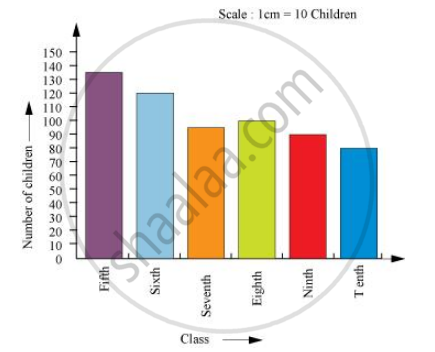Share

# Number of Children in Six Different Classes Are Given Below. Represent the Data on a Bar Graph.How Would You Choose a Scale?Which Class Has the Maximum Number of Children? and the Minimum? - CBSE Class 7 - Mathematics

ConceptUse of Bar Graphs with a Different Purpose - Choosing a Scale

#### Question

Number of children in six different classes are given below. Represent the data on a bar graph.

 Class Fifth Sixth Seventh Eighth Ninth Tenth Number of children 135 120 95 100 90 80

1)  How would you choose a scale?

a) Which class has the maximum number of children? And the minimum?

b) Find the ratio of students of class sixth to the students of class eight.

#### Solution1) We will choose a scale as 1 unit = 10 children because we can represent a more clear difference between the number of students of class 7th and that of class 9th by this scale.

2.1)Since the bar representing the number of children for class fifth is the tallest, there are maximum number of children in class fifth. Similarly, since the bar representing the number of children for class tenth is the smallest, there are minimum number of children in class tenth.

2.2) The number of students in class sixth is 120 and the number of students in class eighth is 100.

Therefore, the ratio between the number of students of class sixth and the number of

students of class eighth = 120/100 = 6/5 = 6 : 5

Is there an error in this question or solution?

#### APPEARS IN

NCERT Solution for Mathematics for Class 7 (2018 to Current)
Chapter 3: Data Handling
Ex. 3.30 | Q: 3 | Page no. 73
Solution Number of Children in Six Different Classes Are Given Below. Represent the Data on a Bar Graph.How Would You Choose a Scale?Which Class Has the Maximum Number of Children? and the Minimum? Concept: Use of Bar Graphs with a Different Purpose - Choosing a Scale.
S